# Wage Rate Calculation Walkthrough

## What is Wage Rate?

In order to more accurately estimate the labor costs of a construction project, the estimator should calculate the total cost to the construction company per hour to employ each worker in a crew. This number is the wage rate, and is more than just the hourly wage workers are paid per hour.

### Wage Rate Formula

#### Important!

Some of the numbers provided for various figues (eg. Employer Contribution Rates) may not match the figures for your assignment. This is done intentionally to demonstrate how the calculations are made, but to not do the work for you.

### Wage Rate Calculation Scope of Work

#### Taxable Wages Section

Complete the calculations for each of the following figures (displayed in orange):

• Overtime Wage Rate
• Number Regular Hour Per Year
• Number Overtime Hours Per Year
• Yearly Regular Wage ($) • Overtime Wages Per Year ($)
• Employee 401(k) Yearly Contribution ($) • Total Employer Non-Taxable Contribution Per Year • Total Employee Non-Taxable Withholding Per Year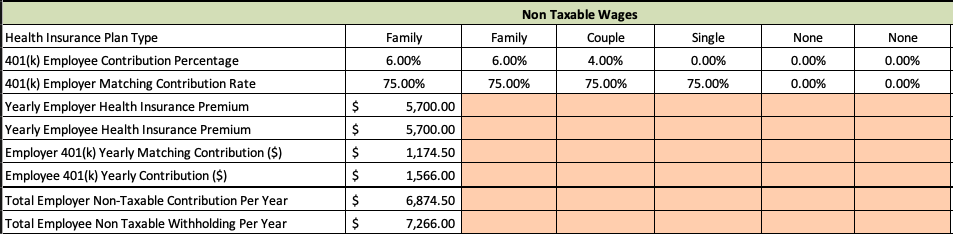Non-taxable Wages section of the Wage Calculator Spreadsheet #### Employer Tax & Insurance Contributions Section Complete the calculations for each of the following figures (displayed in orange): • Total Employer FICA Contribution Per Year • Total Unemployment Premium Per Year (FUTA) • Total Unemployment Premium Per Year (SUTA) • Total Worker's Compensation Premiums Per Year ($)
• Total Liability Premiums Per Year ($) • Total Net Annual Payroll Amount ($)

#### Billable Hours Section

Complete the calculations for each of the following figures (displayed in orange):

• Total Number Hours Paid Per Year
• Total Non-Billable Hours Per Year
• Total Billable Hours Per Year
• Average Hourly Wage Rate

### Wage Rate Calculation Video Walkthroughs

Wage Calculator Assignment Walkthrough (Part 1), 23:28 mins

Wage Calculator Assignment Walkthrough (Part 2), 43:01 mins

### Taxable Wages Calculation

Taxable Wages are all payments and taxable benefits provided to employees. It is calculated using the following steps:

Step 01: Calculate the total regular wages per year by multiplying the hourly wage by the total number of regular hours.

 Example: John Doe is a full-time employee with an hourly rate of $10.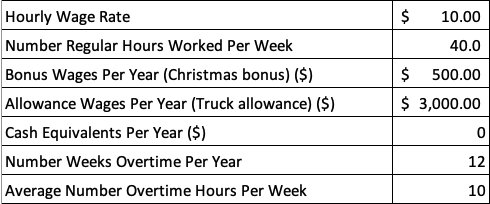John Doe's Taxable Wages information from the Wage Calculator Spreadsheet Hourly Rate =$10 Regular Hours = 2,080 hrs Total Regular Wages = $20,800.00 ($10 x 2,080 hrs)

Step 02: Calculate the overtime rate (1.5 x regular hourly wage).

 Example: John Doe is a full-time employee with an hourly rate of $10. Hourly Rate =$10 Overtime Rate = 1.5 x Hourly Rate Overtime Rate = $15 per hour Step 03: Calculate the total number of overtime hours per year.  Example: John Doe is a full-time employee with an hourly rate of$10. Overtime Weeks = 12 wks/yr Overtime Average Hours = 10 hrs/wk Overtime Hours per Year = 120 hrs

Step 04: Multiply the overtime rate by the total number of overtime hours, then add it to the total regular wages.

 Example: John Doe is a full-time employee with an hourly rate of $10. Overtime Rate =$15 Overtime Hours = 120 hrs Total Overtime Wages = $1,800 ($15 x 120 hrs) Total Wages = $22,600.00 ($20,800 + $1,800) Step 05: Add the value of any bonuses, allowances, and cash equivalents.  Example: John Doe is a full-time employee with an hourly rate of$10. Bonus: Christmas = $500.00 yr Allowance: Truck =$3,000.00 yr Total Bonus & Allowance = $3,500.00 ($500.00 + $3,00.00) Total Taxable Wages =$26,100.00 ($22,600.00 +$3,500.00)

Note that the following items will be pre-populated in any estimation worksheet that includes labor: Worker Hourly Wage, Regular Weekly Hours, Bonuses, Allowances, Overtime Weeks, and Overtime Average Hours.

### Non-Taxable Wages Calculation

Non-taxable Wages are benefits paid to employees that are not subject to payroll tax. They include Health Insurance Premiums from both the employee and employer and Retirement Plan Contributions from both the employee and the employer.

For the purposes of construction estimating, both employee and employer contributions to these benefits is taken into account when calculating Total Non-taxable Wages. To calculate this figure, follow these steps:

Step 01: Calculate the Yearly Employer Health Insurance Premium.

 Example: John Doe is a full-time employee who has the family health insurance plan.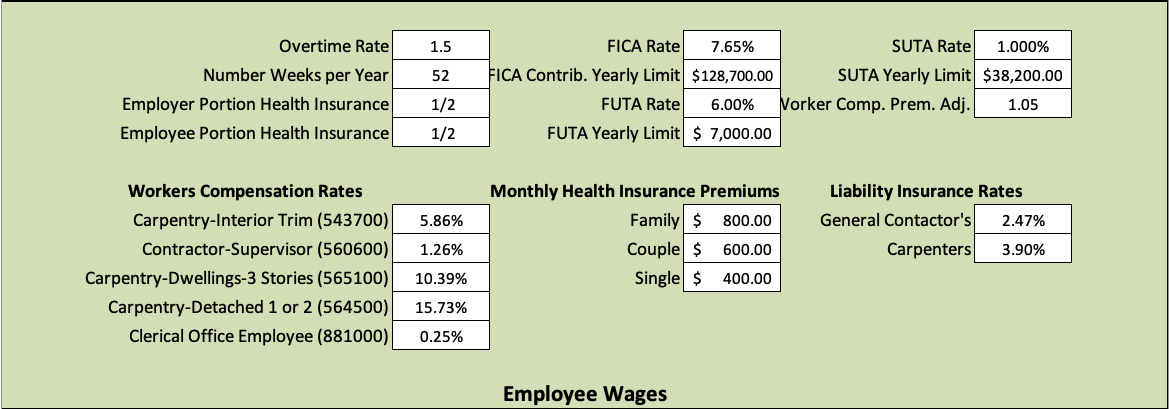Information from the Header of the Wage Calculator Spreadsheet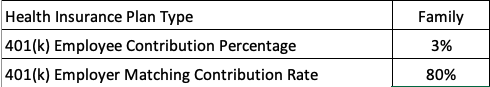John Doe's Non-taxable Wages information from the Wage Calculator Spreadsheet Family Plan Premium (monthly) = $800.00 Family Plan Premium (annual) =$9,600.00 (12 x $800.00) Employer Contribution Percentage = 70% This figure comes from the InsuranceDB tab, and does not match the number used in your own assignment. Yearly Employer Health Insurance Premium =$6,720.00 ($9,600.00 x .7) Step 02: Calculate the Yearly Employee Health Insurance Premium.  Example: John Doe is a full-time employee who has the family health insurance plan. Family Plan Premium (monthly) =$800.00 Family Plan Premium (annual) = $9,600.00 (12 x$800.00) Employee Contribution Percentage = 30% Yearly Employer Health Insurance Premium = $2,880.00 ($9,600.00 x .3)

Step 03: Calculate the Employer 401(k) Yearly Matching Contribution.

 Example: John Doe’s employer matches 80% of his 401(k) contributions. Employee 401(k) Yearly Contribution = $783.00 Employer 401(k) Matching Contribution Rate = 80% Employer 401(k) Yearly Matching Contribution =$626.40

Step 04: Calculate the Employee 401(k) Yearly Contribution.

 Example: John Doe is a full-time employee with a yearly taxable wages total of $26,100.00. John Doe contributes 3% of his taxable wages to a 401(k) retirement account. John Doe Taxable Wages =$26,100.00 John Doe 401(k) Contribution Percentage = 3% Employee 401(k) Yearly Contribution = $783.00 ($26,100 x .03)

Step 05: Calculate the Total Employer Non-taxable Contribution Per Year.

 Example: John Doe is a full-time employee. Yearly Employer Health Insurance Premium = $6,720.00 Employer 401(k) Yearly Matching Contribution =$626.40 Total Employer Non-taxable Wages per Year = $7,346.40 ($6,720.00 + $626.40) Step 06: Calculate the Total Employee Non-taxable Withholding Per Year.  Example: John Doe is a full-time employee. Yearly Employer Health Insurance Premium =$2,880.00 Employee 401(k) Yearly Contribution = $783.00 Total Employee Non-taxable Withholding per Year =$3,663.00 ($2,880.00 +$783.00)

Note that the following items will be pre-populated in any estimation worksheet that includes labor: Health Insurance Plan, 401(k) Employee Contribution Rate, and 401(k) Employer Matching Rate.

### Employer Tax Calculation

Step 01: Look up the current year FICA Earnings Limit on the Social Security Administration’s website (ssa.gov).

 Example: John Doe is a full-time employee.Information from the Header of the Wage Calculator Spreadsheet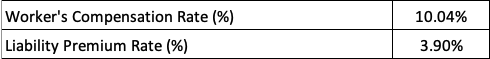John Doe's Employer Tax & Insurance Contributions information from the Wage Calculator Spreadsheet John’s 2018 Total Taxable Wages = $26,100.00 2018 FICA Earnings Limit =$128,400.00 John’s wages are below the limit, so all of his taxable wages will be subject to FICA taxes.

Step 02: Calculate the Wages Subject to FICA Contributions, which are equal to total taxable wages - yearly employee health insurance premium - yearly employee 401(k) contribution.

 Example: John Doe is a full-time employee. Total Taxable Wages = $26,100.00 Yearly Employee Health Insurance Premium =$2,880.00 Yearly Employee 401(k) Contribution = $783.00 Wages Subject to FICA Contributions =$22,437.00 ($26,100.00 -$2,880.00 - $783.00) Step 03: Calculate Total FICA Contribution (15.3%).  Example: John Doe is a full-time employee. Wages Subject to FICA Contributions =$22,437.00 Total FICA Contributions = $3,432.86 ($22,437.00 x .153)

Step 04: Calculate Employer FICA Contribution (50% of total contribution).

 Example: John Doe is a full-time employee. Total FICA Contributions = $3,432.86 Employer FICA Contributions =$1,716.43 ($3,432.86 x .5) ### Employer Insurance Contributions Calculation By law, employers are required by law to carry Federal Unemployment Insurance (FUTA), State Unemployment Insurance (SUTA), and Workers’ Compensation Insurance. Employers should also carry Liability Insurance to shield them from claims for bodily injury, property damage, or loss that may arise as a result of failing to use reasonable care in conducting business. The cost of these insurance premiums contribute to the cost of labor, and should be accounted for when completing a construction estimate. They are calculated as follows: Step 01: Look up the current year FUTA Tax Rate on the Internal Revenue Service’s website (irs.gov).  Example: John Doe is a full-time employee.Information from the Header of the Wage Calculator Spreadsheet John’s 2018 Total Taxable Wages =$26,100.00 2018 FUTA Tax Rate = 6.00% on the first $7,000.00 of wages John’s wages are over$7,000.00, so the FUTA Premium will be calculated for $7,000.00. Step 02: Calculate the Total FUTA Premium.  Example: John Doe is a full-time employee. John’s 2018 Total Taxable Wages =$26,100.00 2018 FUTA Tax Rate = 6.00% Total FUTA Premium = $420.00 ($7,000 x .06)

Step 03: Look up the current year SUTA Tax Rate on the Idaho Department of Labor’s website (labor.idaho.gov).

 Example: John Doe is a full-time employee. John’s 2018 Total Taxable Wages = $26,100.00 2018 SUTA Tax Rate = 1.00% on the first$38,200.00 of wages John’s wages are below $38,200.00, so the SUTA Premium will be calculated based on his total taxable wages. Step 04: Calculate the Total SUTA Premium.  Example: John Doe is a full-time employee. John’s 2018 Total Taxable Wages =$26,100.00 2018 SUTA Tax Rate = 1.00% Total SUTA Premium = $261.00 ($26,100 x .01)

Step 05: Identify the Workers’ Compensation Rate for each employee, and the Workers’ Compensation Premium Adjustment (Mod Factor). These will both be available in any estimating worksheet for labor.

 Example: John Doe is a full-time employee. John’s Workers’ Compensation Rate = 10.04% Employer’s Mod Factor = 1.05%

Step 06: Calculate the Workers’ Compensation Premium before Mod Factor.

 Example: John Doe is a full-time employee. John’s 2018 Total Taxable Wages = $26,100.00 John’s Workers’ Compensation Rate = 10.04% Workers’ Compensation Premium (before Mod Factor) =$2,620.44 ($26,100 x .1004) Step 07: Calculate the Workers’ Compensation Premium after Mod Factor.  Example: John Doe is a full-time employee. Workers’ Compensation Premium (before Mod Factor) =$2,620.44 Mod Factor = 1.05% Workers’ Compensation Premium = $2,751.46 ($26,100 x 1.05)

Step 08: Identify the Liability Premium Rate for each employee. These will be available in any estimating worksheet for labor.

 Example: John Doe is a full-time employee. Liability Premium Rate = 3.9%

Step 09: Calculate the Liability Premium.